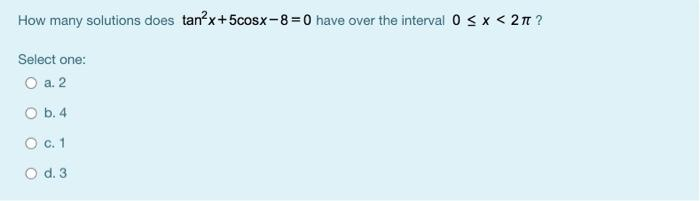# Question Solved1 AnswerHow many solutions does tan?x+5cosx-8=0 have over the interval 0 < x < 211? Select one: O a. 2 O b.4 O c. 1 O d. 3Transcribed Image Text: How many solutions does tan?x+5cosx-8=0 have over the interval 0 < x < 211? Select one: O a. 2 O b.4 O c. 1 O d. 3
More
Transcribed Image Text: How many solutions does tan?x+5cosx-8=0 have over the interval 0 < x < 211? Select one: O a. 2 O b.4 O c. 1 O d. 3